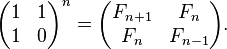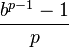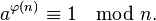# Numeric algorithms

#### sal/algo/numerics.h

 modular_pow modular exponentiation int_pow integer exponentiation fibonacci nth fibonacci number make_cyclic create a cyclic number from 1/prime in given base cyclic_length length of cyclic number from 1/prime in given base, 0 if acyclic is_pow checks if guess is a power of base is_square checks if number is a perfect square gcd greatest common denominator (binary) gcd_euclidean Euclidean algorithm for gcd gcd_alt Another way for expressing the Euclidean algorithm totient number of integers less than n that is relatively prime with n mul matrix chain multiplication ordering factorize prime factorization of smooth numbers factorize_rough prime factorization of numbers with large prime factors num_factors total number of factors (including composites) sum_factors sum of all factors (including composites)

### Exponentiation ¶

Declaration

Example

Discussion

The general approach is to exponentiate by squaring the base and reducing the exponent to at most half each time. This gurantees completion after `Θ(lg(exponent))` computations. Some more examples here.

### Fibonacci ¶

Declaration

Parameters

 n nth fibonacci number, sequence starting: (n=0)0, 1, 1..

Example

Discussion

Since exponentiation can be done in `Θ(lg(n))` time, expanding out clever matrices also shares that time complexity. Because the fibonacci sequence can be defined recursively as a linear combination of previous terms, such a matrix exists (the companion matrix), and is:### Cyclic numbers ¶

Declaration

Parameters

 base number base prime prime that does not divide base

Example

Discussion

Cyclic numbers are related to repeating decimals, from which they can be generated in a given base b with prime p using the relationThey can be constructed by computing the digits of `1/p` in base b by long division and collecting the digits.

### Integer power ¶

Declaration

Example

Discussion

Through divisions, checks whether guess is an integer power of base.

### Perfect square ¶

Declaration

Example

Discussion

Used in tight loops of many number theory problems. Algorithm is written by maartinus from stackoverflow.

### Greatest Common Denominator ¶

Declaration

Parameters

 a integer (can be negative) b integer (can be negative)

Example

Discussion

Often used to solve combination problems. The binary optimization is used because gcd's common usage in time critical operations. The alternative versions are much simpler and easier to memorize.

### Euler's Totient ¶

Declaration

Parameters

 n number to find the totient of

Example

Discussion

Number of positive integers less than n that is relatively prime to n.
`1 < k < n such that gcd(k,n) == 1`

It is multiplicative, so `phi(a*b) == phi(a) * phi(b)`

One application is in Euler's theorem: that a and n are relatively prime iffWith applications here.

### Matrix Chain Multiplication ¶

Declaration

Example

Discussion

`Θ(n^3)` work is done optimally parenthesize the multiplications using dynamic programming. This order affects the number of operations required; using Wikipedia's example:

suppose A is a 10 × 30 matrix, B is a 30 × 5 matrix, and C is a 5 × 60 matrix. Then,
(AB)C = (10×30×5) + (10×5×60) = 1500 + 3000 = 4500 operations
A(BC) = (30×5×60) + (10×30×60) = 9000 + 18000 = 27000 operations.

### Integer Factorization ¶

Declaration

Example

Discussion

Factorizing in polynomial time is still an open problem.
Smooth numbers are ones that have small prime factors, while rough numbers factor into large primes. Trial division is used to factorize both, with the difference being the divisor sequence for smooth numbers being that of odd numbers (2, 3, 5, 7, 9,...), while rough numbers is that of generated primes (2, 3, 5, 7, 11,...). Trial division is very fast for practical encounters.